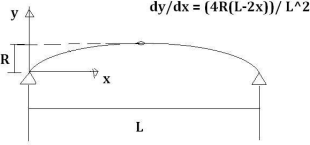Search IntMath
Close

# Proof of equation [Solved!]

### My question

hi,
i need the proof of the following equation:

y = (4Rx(L-2x)) / L^2

I shall be very thankful to you.

### Relevant page

Applications of Differentiation

### What I've done so far

Tried to work out the proof, but couldn't

X

hi,
i need the proof of the following equation:

y = (4Rx(L-2x)) / L^2

I shall be very thankful to you.
Relevant page

<a href="/applications-differentiation/applications-of-differentiation-intro.php">Applications of Differentiation</a>

What I've done so far

Tried to work out the proof, but couldn't

## Re: Proof of equation

Hello Hasham

But there is nothing to "prove" in the equation you wrote?

X

Hello Hasham

But there is nothing to "prove" in the equation you wrote?

## Re: Proof of equation

hi,
actualy i wanted to know the procedure of the proof of that equation.

X

hi,
actualy i wanted to know the procedure of the proof of that equation.

## Re: Proof of equation

Hi again Hasham

Like all mathematics equations, you need to state the situation and the meaning of each of the variables.

What does y represent here? What are R and L?

Let me know these and I may be able to help you.

X

Hi again Hasham

Like all mathematics equations, you need to state the situation and the meaning of each of the variables.

What does y represent here? What are R and L?

Let me know these and I may be able to help you.

## Re: Proof of equation

hi, here is the information:

y= length of parabola of a structure
R = vertical distance b/w highest point if parabola and supports
L = length of span lets say of a bridge
x= x coordinate of any point

basicaly the equation is to find out the length of a parabola lets say of a bridge or something.

X

hi, here is the information:

y= length of parabola of a structure
R = vertical distance b/w highest point if parabola and supports
L = length of span lets say of a bridge
x= x coordinate of any point

basicaly the equation is to find out the length of a parabola lets say of a bridge or something.

## Re: Proof of equation

Hello Hasham

Ah, I see. "Length of parabola" possibly means the curve length, for which you need integration, not differentiation. See the last example on this page:

5. Integration: Other Trigonometric Forms

Is that what you mean?

I think it best if you draw a diagram then we'll both be talking about the same thing.

Another point: "y= length of parabola of a structure" is not quite correct here - actually, y will be the height of the parabola x units from the origin.

X

Hello Hasham

Ah, I see. "Length of parabola" possibly means the curve length, for which you need integration, not differentiation. See the last example on this page:

<a href="/methods-integration/5-integration-other-trigonometric-forms.php">5. Integration: Other Trigonometric Forms</a>

Is that what you mean?

I think it best if you draw a diagram then we'll both be talking about the same thing.

Another point: "y= length of parabola of a structure" is not quite correct here - actually, y will be the height of the parabola x units from the origin.

## Re: Proof of equation

hi,
now i attached the fig. i have to explain the things as posible to me.
w8ing for the solution.X

hi,
now i attached the fig. i have to explain the things as posible to me.
w8ing for the solution.

<img src="/forum/uploads/parabola310.png" width="310" height"145" alt="parabola" />

## Re: Proof of equation

Hmmm - your diagram is fine (it represents the parabola you were talking about at the beginning), but you now have dy/dx at the beginning, which is a bit confused.

To find the arc length of a parabola, you need integration, not differentiation.

So let's clarify - is your question asking for the arc length of this parabola?

X

Hmmm - your diagram is fine (it represents the parabola you were talking about at the beginning), but you now have dy/dx at the beginning, which is a bit confused.

To find the arc length of a parabola, you need integration, not differentiation.

So let's clarify - is your question asking for the arc length of this parabola?

## Re: Proof of equation

Yes, I need to find the length of the parabola.

X

Yes, I need to find the length of the parabola.

## Re: Proof of equation

OK, please post your actual question (which is not asking for a proof, nor for differentiating the expression) in the Applications of Integration forum.

X

OK, please post your actual question (which is not asking for a proof, nor for differentiating the expression) in the <a href="/forum/applications-integration-30/">Applications of Integration</a> forum.

You need to be logged in to reply.

## Related Applications of Differentiation questions

• curve sketching [Solved!]
Wrote you earlier today. Confusion might come from Example 4. Three lines before...
• Concavity [Solved!]
In curve sketching with differentiation, the general curves section, there are no points of inflection...
• Practical application - normal to a curve? [Solved!]
Hi there. First off, I just wanted to say a huge thank you for the...
• Rate of change: conical tank [Solved!]